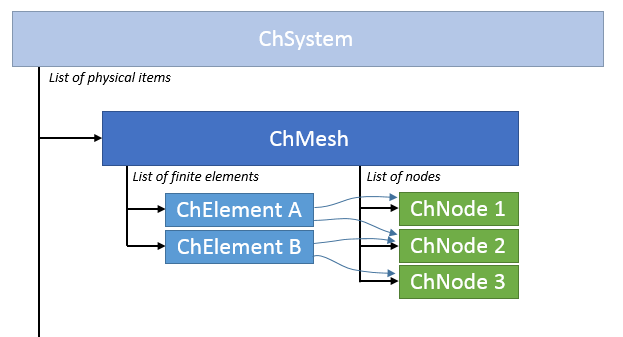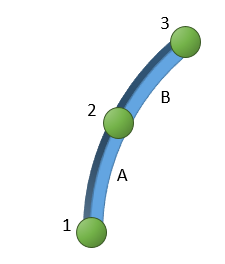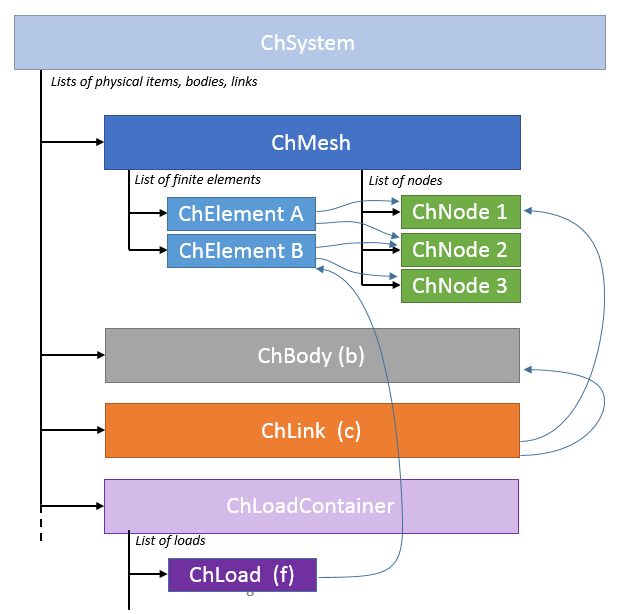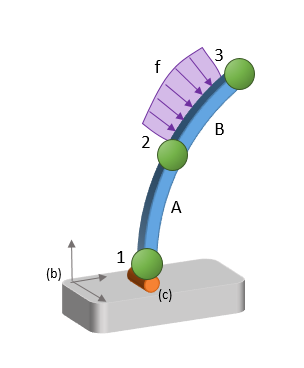Finite Elements

This part discusses the Chrono FEA module.

Finite elements can be used in Chrono to model flexible parts.

Different types of finite elements are supported.

• solid volume elements like tetrahedrons can be used to model parts with arbitrary geometry, such as metal shapes, plastic molds, biomechanical tissues like muscles, etc.
• surface volume elements like shells can be used to model thin parts, like tires, airbags, etc.
• beam elements can be used to model cables, wires, thin shafts, blades of wind turbines, etc.

For most of these elements we support large displacements. This allows, for instance, the simulation of problems like rubber structures undergoing large deflections, airplane taking complex manouvers in space, blades of wind turbines with large rotations and other geometric non-linearities. Of course linearized small-displacement analysis is supported too, as a subcase.

Detailed documentation on nodes and elements can be found in these pages:

# Finite element data structures

Data structures for a FEA model are organized in this way:One can see that the main ingredients are

Note, however, that one can also use some of the chrono::ChLink objects to constraint nodes to other nodes or to chrono::ChBody objects. Also, some chrono::ChLoad objects like forces, torques, pressures, can be added. In the following example we show the data structures for a case with two elements, a constraint, a load:In general:

• A mesh is a container for nodes and elements
• Multiple meshes are allowed in a single system
• A node has degrees of freedom (xyz, rotations, etc.).
• Look at the list of nodes.
• An element connects N nodes.
• Initialize the elements by telling which node are connected with SetNodes()
• Set a material property to the element by using SetMaterial()
• Look at the list of elements.

# How to create a FEA model

Creating/Setting up a finite element mesh typically involves the following steps:

1. Create the chrono::fea::ChMesh, and add it to a chrono::ChSystem
2. Create some nodes
3. Create a material for elements, if needed
4. Create some elements between nodes

The following example illustrates it.

## 1) Create a ChMesh

• Create the mesh (do this using std::make_shared, so it will be handled by a shared pointer, and you will not worry about deleting it)
• Add the mesh to a physical system:
// The physical system: it contains all physical objects.
ChSystem my_system;
// Create a mesh, that is a container for groups
// of elements and their referenced nodes.
auto my_mesh = std::make_shared<ChMesh>();
// Remember to add the mesh to the system!

## 2) Create some nodes

• Create nodes, using shared pointers.
• Note: usually node positions are set as parameters in their constructors
• Add nodes to the mesh
// Create some point-like nodes with x,y,z degrees of freedom
// While creating them, also set X0 undeformed positions.
auto mnode1 = std::make_shared<ChNodeFEAxyz>(ChVector<>(0, 0, 0));
auto mnode2 = std::make_shared<ChNodeFEAxyz>(ChVector<>(0, 0, 1));
auto mnode3 = std::make_shared<ChNodeFEAxyz>(ChVector<>(0, 1, 0));
auto mnode4 = std::make_shared<ChNodeFEAxyz>(ChVector<>(1, 0, 0));
// Remember to add nodes and elements to the mesh!
• Set node properties, if you need.
• Ex. most node classes provide a way to apply a local force (or even a torque if they have rotational DOFs) by using SetForce() , an easier alternative to using ChLoad classes, if the force is constant.
• Here you may want also to attach an optional local point-mass using SetMass() for the nodes; otherwise the default mass for FEA nodes is zero, as mass is mostly added by finite elements.
// For example, set some non-zero mass concentrated at the nodes
mnode1->SetMass(0.01);
mnode2->SetMass(0.01);
// For example, set an applied force to a node:
mnode2->SetForce(ChVector<>(0, 5, 0));

## 3) Create a material

• Create the material, using shared pointers.
• Note that not all elements need a material, for instance ChElementSpring has no material.
• A single material can be shared between multiple elements.
// Create a material, that will be assigned to each element,
auto mmaterial = std::make_shared<ChContinuumElastic>();
// …and set its parameters
mmaterial->Set_E(0.01e9); // rubber 0.01e9, steel 200e9
mmaterial->Set_v(0.3);

## 4) Create FEA elements

• Create the elements, using shared pointers.
• Add the elements to the mesh.
• Assign nodes (which you created before) to the element.
• Assign material(s) to the elements.
// Create the tetrahedron element,
auto melement1 = std::make_shared<ChElementTetraCorot_4>();
// Remember to add elements to the mesh!